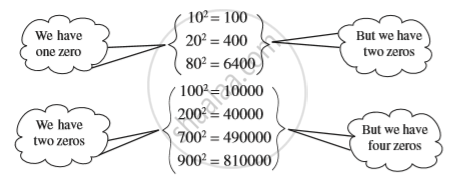Share

# Properties of Square Numbers

Course

#### notes

Following table shows the squares of numbers from 1 to 20.

 Number Square Number Square 1 1 11 121 2 4 12 144 3 9 13 169 4 16 14 196 5 25 15 225 6 36 16 256 7 49 17 289 8 64 18 324 9 81 19 361 10 100 20 400

We observ that the square number ends with either 0 or 1 or 4 or 5 or 6 or 9 at its unit's place. We say that a square number always as will either 0 , 1 , 4 , 5,6 and 9T it's unit's place .
The following square numbers end with digit 1.

 Square Number 1 1 81 9 121 11 361 19 441 21

We say that if a number has 1 or 9 in the units place, then it’s square ends in 1.
Let us consider square numbers ending in 6.

 Square Number 16 4 36 6 196 14 256 16

when a square number ends in 6, the number whose square it is, will have either 4 or 6 in unit’s place.
Consider the following numbers and their squares.We observe  that  a square number always has an even number of zeros at its end. A number with odd number of zeros is never a square of any number.

### Shaalaa.com

Properties of Square Numbers [00:06:31]
S
0%

S